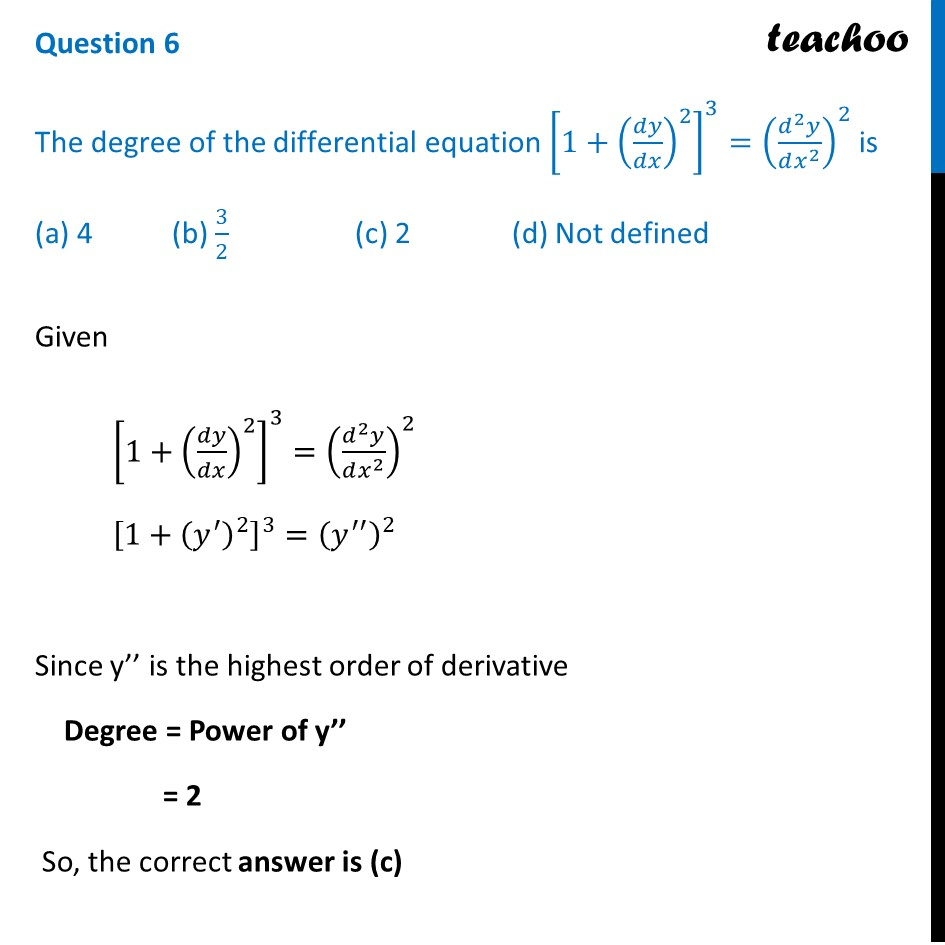CBSE Class 12 Sample Paper for 2024 Boards

Class 12
Solutions of Sample Papers and Past Year Papers - for Class 12 Boards

## (a) 4           (b) 3/2                   (c) 2              (d) Not defined

This question is similar to Question-2 CBSE-Class 12 Sample Paper for 2022-Boards For Term-2Learn in your speed, with individual attention - Teachoo Maths 1-on-1 Class

### Transcript

Given [1+(𝑑𝑦/𝑑𝑥)^2 ]^3=((𝑑^2 𝑦)/(𝑑𝑥^2 ))^2 [1+(𝑦^′ )^2 ]^3=(𝑦^′′ )^2 Since y’’ is the highest order of derivative Degree = Power of y’’ = 2 So, the correct answer is (c)